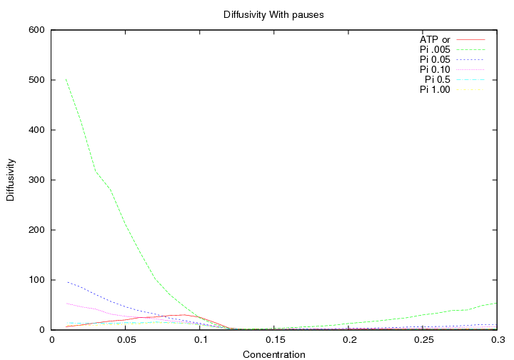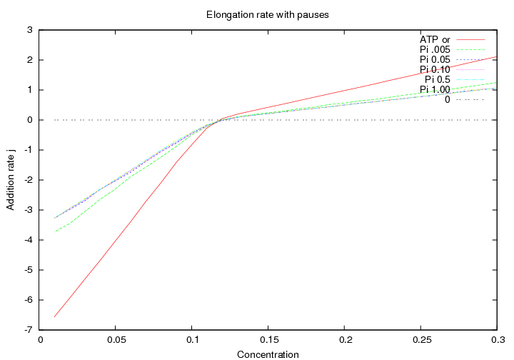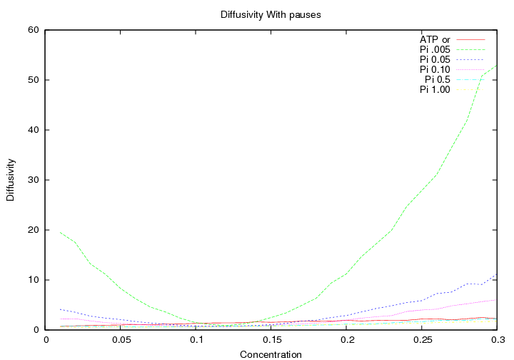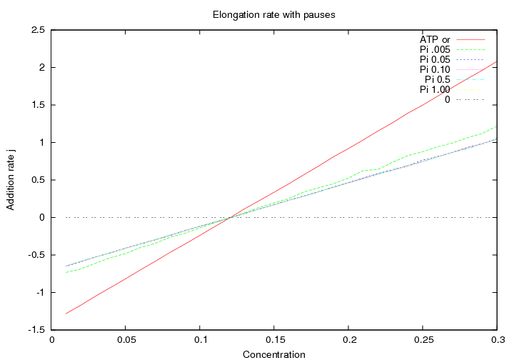posted on: 2008-06-10 10:21:22
This is a short summary of my current pause results before I proceed. I want to make sure whether the results make sense.

>The main change in my algorithm has to do with time. Before it would update the time and check to see if it had taken to long, but it would perform the action regards. This means that the combined rate would have to correspond to a time interval smaller than my time interval. Now each filament keeps track of its own time. So that problem is gone.

My results still seem a little different. So first here are the graphs of the values from the PNAS paper.And These are the results when I change the values to all have the same depolymerization constant. I also set the hydrolysis rate and phosphate release rate to zero so that the only thing is polymerization, depolymerization and pauses.So the lsat two graphs look symmetric, wrt the rate. The first two could be correct considering the rates at the two ends are -7 and 2, which is a little over a factor of three. And the diffusivity is going as j^2, so 3 squared is ~10 and the two ends appear to be a factor of ten different.